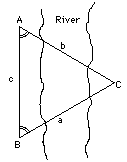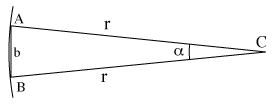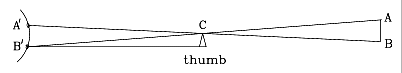# (8b) ParallaxIndex5c. Coordinates6. The Calendar6a. Jewish Calendar7.Precession8. The Round Earth8a. The Horizon8b. Parallax8c. Moon dist. (1)8d. Moon dist. (2)9a. Earth orbits Sun?9b. The Planets9c. Copernicus
to Galileo10. Kepler's Laws10a. Scale of Solar Sys.11a. Ellipses
and First Law## "Pre-Trigonometry"

Section M-7 describes the basic problem of trigonometry (drawing on the left): finding the distance to some far-away point C, given the directions at which C appears from the two ends of a measured baseline AB. This problem becomes somewhat simpler if:
1. The baseline is perpendicular to the line from its middle to the object, so that the triangle ABC is symmetric. We will denote its side by r:

AC = BC = r

2. The length c of the baseline AB is much less than r. That means that the angle α between AC and BC is small; that angle is known as the parallax of C, as viewed from AB.

3. We do not ask for great accuracy, but are satisfied with an approximate value of the distance--say, within 1%.
The method presented here was already used by the ancient Greeks more than 2000 years ago. They knew that the length of a circle of radius r was 2πr, where π (a modern notation, not one of the Greeks, even though π is part of their alphabet) stands for a number a little larger than 3, approximately

π = 3.14159...

(The Greek mathematician Archimedes derived π to about 4-figure accuracy, though he expressed it differently, since decimal fractions only appeared in Europe some 1000 years later.)

 Draw a circle around the point C, with radius r, passing through A and B (drawing above). Since the angle α is so small, the length of the straight-line "baseline" b (drawing on the right; distance AB renamed) is not much different from the arc of the circle passing A and B. Let us assume the two are the same (that is the approximation made here). The length of a circular arc is proportional to the angle it covers, and sinceb covers an angle    α
2π r covers an angle 360°

we get

2π r = (360°/ α) b

and dividing by 2π

r = (360°/2 π α) b

Therefore, if we know b, we can deduce r. For instance, if we know that α = 5.73°, 2 π α = 36° and we get (approximately)

r = 10 b

## Estimating distance outdoors

This method can be used in a way useful to hikers and scouts. Suppose you want to estimate the distance to some far-away landmark--e.g. a building, tree or water tower.

The drawing shows a schematic view of the situation from above (not to scale). To estimate the distance to the landmark A, you do the following:Stretch your arm forward and extend your thumb, so that your thumbnail faces your eyes. Close one eye (A') and move your thumb so that, looking with your open eye (B'), you see your thumbnail covering the landmark A.   Then open the eye you had closed (A') and close the one (B') with which you looked before, without moving your thumb. It will now appear that your thumbnail has moved: it is no longer in front of landmark A, but in front of some other point at the same distance, marked as B in the drawing.   Estimate the true distance AB, by comparing it to the estimated heights of trees, widths of buildings, distances between power-line poles, lengths of cars etc. The distance to the landmark is 10 times the distance AB.   Why does this work? Because even though people vary in size, the proportions of the average human body are fairly constant, and for most people, the angle between the lines from the eyes (A',B') to the outstretched thumb is about 6°, close enough to the value 5.73° for which the ratio 1:10 was found in an earlier part of this section.   That angle is the parallax of your thumb, viewed from your eyes. The triangle A'B'C has the same proportions as the much larger triangle ABC, and therefore, if the distance B'C to the thumb is 10 times the distance A'B' between the eyes, the distance AC to the far landmark is also 10 times the distance AB.

## How far to a Star?

When estimating the distance to a very distant object, our "baseline" between the two points of observation better be large, too. The most distant objects our eyes can see are the stars, and they are very far indeed: light which moves at 300,000 kilometers (186,000 miles) per second, would take years, often many years, to reach them. The Sun's light needs 500 seconds to reach Earth, a bit over 8 minutes, and about 5 hours to reach the the distant planet
Pluto. A "light year" is about 1600 times further, an enormous distance.

The biggest baseline available for measuring such distances is the diameter of the Earth's orbit, 300,000,000 kilometers. The Earth's motion around the Sun makes it move back and forth in space, so that on dates separated by half a year, its positions are 300,000,000 kilometers apart. In addition, the entire solar system also moves through space, but that motion is not periodic and therefore its effects can be separated.

And how much do the stars shift when viewed from two points 300,000,000 km apart? Actually, very, very little. For many years astronomers struggled in vain to observe the difference. Only in 1838 were definite parallaxes measured for some of the nearest stars--for Alpha Centauri by Henderson from South Africa, for Vega by Friedrich von Struve and for 61 Cygni by Friedrich Bessel.

Such observations demand enormous precision. Where a circle is divided into 360 degrees (360°), each degree is divided into 60 minutes (60')--also called "minutes of arc" to distinguish them from minutes of time--and each minute contains 60 seconds of arc (60"). All observed parallaxes are less than 1", at the limit of the resolving power of even large ground-based telescopes.

In measuring star distances, astronomers frequently use the parsec, the distance to a star whose yearly parallax is 1"--one second of arc. One parsec equals 3.26 light years, but as already noted, no star is that close to us. Alpha Centauri, the sun-like star nearest to our solar system, has a distance of 4.3 years and a parallax of 0.75".

Alpha Centauri is not a name, but a designation. Astronomers designate stars in each constellation by letters of the Greek alphabet--alpha, beta, gamma, delta and so forth, and "Alpha Centauri" means the brightest star in the constellation of Centaurus, located high in the southern skies. You need to be south of the equator to see it well.

Questions from Users:         ***   Are all stars we see suns?

Next Stop: #8c. How Distant is the Moon?--1

Author and Curator:   Dr. David P. Stern
Mail to Dr.Stern:   stargaze("at" symbol)phy6.org .

Last updated: 1 April 2014